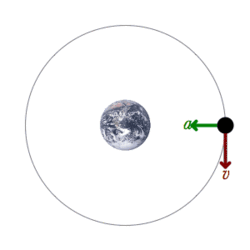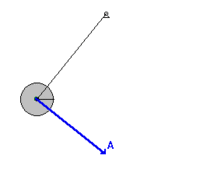# Mechanical energy

﻿
Mechanical energyAn example of a mechanical system: A satellite is orbiting the Earth only influenced by the conservative gravitational force and the mechanical energy is therefore conserved. The satellite is accelerated towards the Earth with an acceleration perpendicular to the orbit. This acceleration is represented by a green acceleration vector and the velocity is represented by a red velocity vector. Though the velocity is constantly changed with the direction of the vector because of the acceleration vector, the speed of the satellite is not since the magnitude of the velocity vector remains unchanged.

In physics, mechanical energy is the sum of potential energy and kinetic energy present in the components of a mechanical system. It is the energy associated with the motion and position of an object. The law of conservation of energy states that in an isolated system that is only subject to conservative forces, like the gravitational force, the mechanical energy is constant. If an object is moved in the opposite direction of a conservative net force, the potential energy will increase and if the speed (not the velocity) of the object is changed, the kinetic energy of the object is changed as well. In all real systems, however, non-conservative forces, like frictional forces, will be present, but often they are of negligible values and the mechanical energy's being constant can therefore be a useful approximation. In elastic collisions, the mechanical energy is conserved but in inelastic collisions, some mechanical energy is converted into heat. The equivalence between lost mechanical energy and an increase in temperature was discovered by James Prescott Joule.

Many modern devices, such as the electric motor or the steam engine, are used today to convert mechanical energy into other forms of energy, e.g. electrical energy, or to convert other forms of energy, like heat, into mechanical energy.

## General

Energy is a scalar quantity and the mechanical energy of a system is the sum of the potential energy which is measured by the position of the parts of the system, and the kinetic energy which is also called the energy of motion:$E_{mechanical}=U+K\,$

The law of conservation of mechanical energy states that if a body or system is subjected only to conservative forces, the total mechanical energy of that body or system remains constant. The difference between a conservative and a non-conservative force is that when a conservative force moves an object from one point to another, the work done by the conservative force is independent of the path. On the contrary, when a non-conservative force acts upon an object, the work done by the non-conservative force is dependent of the path.

The potential energy, U, depends on the position of an object subjected to a conservative force. It is defined as the object's ability to do work and is increased as the object is moved in the opposite direction of the direction of the force.[nb 1] If F represents the conservative force and x the position, the potential energy of the force between the two positions x1 and x2 is defined as the negative integral of F from x1 to x2:$U = - \int\limits_{x_1}^{x_2} \vec{F}\cdot d\vec{x}$

The kinetic energy, K, depends on the speed of an object and is the ability of a moving object to do work on other objects when it collides with them.[nb 2] It is defined as one half the product of the object's mass with the square of its speed, and the total kinetic energy of a system of objects is the sum of the kinetic energies of the respective objects:$K={1 \over 2}mv^2$

## Conservation and interconversion of energy

Of the three great conservation laws of classical mechanics, the conservation of energy is regarded as the most important.[nb 3] According to this law, the mechanical energy of an isolated system remains constant in time, as long as the system is free of all frictional forces, including eventual internal friction from collisions of the objects of the system. In any real situation, frictional forces and other non-conservative forces are always present, but in many cases their effects on the system are so small that the principle of conservation of mechanical energy can be used as a fair approximation. Though energy cannot be created nor destroyed in an isolated system, it can be internally converted to any other form of energy.A swinging pendulum with the velocity vector (green) and acceleration vector (blue). The magnitude of the velocity vector, the speed, of the pendulum is greatest in the vertical position and the pendulum is farthest from Earth in its extreme positions.

Thus, in a mechanical system like a swinging pendulum subjected to the conservative gravitational force where frictional forces like air drag and friction at the pivot are negligible, energy passes back and forth between kinetic and potential energy but never leaves the system. The pendulum reaches greatest kinetic energy and least potential energy when in the vertical position, because it will have the greatest speed and be nearest the Earth at this point. On the other hand, it will have its least kinetic energy and greatest potential energy at the extreme positions of its swing, because it has zero speed and is farthest from Earth at these points. However, when taking the frictional forces into account, the system loses mechanical energy with each swing because of the work done by the pendulum to oppose these non-conservative forces.

That the loss of mechanical energy in a system always resulted in an increase of the system's temperature has been known for a long time, but it was the amateur physicist James Prescott Joule who first experimentally demonstrated how a certain amount of work done against friction resulted in a definite quantity of heat which should be conceived as the random motions of the particles that matter is composed of.  This equivalence between mechanical energy and heat is especially important when considering colliding objects. In an elastic collision, mechanical energy is conserved; i.e. the sum of the kinetic energies of the colliding objects is the same before and after the collision. After an inelastic collision, however, the total mechanical energy of the system will have changed. Usually, the total mechanical energy after the collision is smaller than the initial total mechanical energy and the lost mechanical energy is converted into heat. However, the total mechanical energy can be greater after an inelastic collision if for example the collision causes an explosion which converts chemical energy into mechanical energy. In inelastic collisions, the smaller particles of which the colliding objects consist are shaken up and rattle around. These small-scale motions are perceived as an increase in heat and need kinetic energy which must be taken from the large-scale motion of the objects that are observed directly. Thus, the total energy of the system remains unchanged though the mechanical energy has been changed.

## Conversion

Today, many technological devices converts mechanical energy into other forms of energy or vice versa. These devices can be placed in these categories:

## Distinguished from other types

The classification of energy into different types often follows the boundaries of the fields of study in the natural sciences.

Wikimedia Foundation. 2010.

### Look at other dictionaries:

• mechanical energy — Sum of a system s kinetic energy (KE) and potential energy (PE). Mechanical energy is constant in a system that experiences no dissipative forces such as friction or air resistance. For example, a swinging pendulum that experiences only… …   Universalium

• mechanical energy — noun energy in a mechanical form • Hypernyms: ↑energy, ↑free energy • Hyponyms: ↑kinetic energy, ↑K.E., ↑potential energy, ↑P.E. * * * mechanical energy …   Useful english dictionary

• mechanical energy — mechaninė energija statusas T sritis Standartizacija ir metrologija apibrėžtis Mechaninės sistemos kinetinės ir potencinės energijos suma. atitikmenys: angl. mechanical energy vok. mechanische Energie, f rus. механическая энергия, f pranc.… …   Penkiakalbis aiškinamasis metrologijos terminų žodynas

• mechanical energy — mechaninė energija statusas T sritis chemija apibrėžtis Kinetinės ir potencinės energijos suma. atitikmenys: angl. mechanical energy rus. механическая энергия …   Chemijos terminų aiškinamasis žodynas

• mechanical energy — mechaninė energija statusas T sritis fizika atitikmenys: angl. mechanical energy vok. mechanische Energie, f rus. механическая энергия, f pranc. énergie mécanique, f …   Fizikos terminų žodynas

• mechanical energy — mechaninė energija statusas T sritis Energetika apibrėžtis Mechaninio medžiagų judėjimo ir medžiagų ar jų dalių sąveikos energija. Mechaninė kūnų sistemos energija – kinetinės ir potencinės energijų suma. atitikmenys: angl. mechanical energy vok …   Aiškinamasis šiluminės ir branduolinės technikos terminų žodynas

• mechanical energy — noun The sum of the potential energy and kinetic energy of a body or system …   Wiktionary

• Energy storage — is the storing of some form of energy that can be drawn upon at a later time to perform some useful operation. A device that stores energy is sometimes called an accumulator. All forms of energy are either potential energy (eg. chemical,… …   Wikipedia

• Energy harvesting — (also known as Power harvesting or energy scavenging ) is the process by which energy is captured and stored. Frequently this term is applied when speaking about small autonomous devices, like those used in sensor networks. A variety of different …   Wikipedia

• Energy —   The capability of doing work; different forms of energy can be converted to other forms, but the total amount of energy remains the same.   This is broadly defined as the capability of doing work. In the electric power industry, energy is more… …   Energy terms